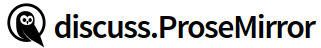# Track Changes w/ marks

I’ve done a lot of research about how to use ProseMirror to track deletions (e.g. `prosemirror-changeset`, fiduswriter, etc.), but I haven’t found anything that works how I think track changes should work.

I’ve been trying to use `rebaseSteps` from `prosemirror-collab` along with `inserted` and `deleted` marks to mark how the state has changed over a series of steps. The code below seems to work fine for changes within a paragraph, but when there is a `ReplaceStep` that spans paragraphs and lists items, I run into issues like “Inconsistent open depths”.

My thinking was that if looped through the original steps, then I could add additional steps to each of the original steps and then rebase the remaining original steps. This seems similar to how `prosemirror-collab` works where the extra steps I’m adding are the steps provided in `receiveTransaction` here: https://github.com/ProseMirror/prosemirror-collab/blob/master/src/collab.js#L115.

There must either be something wrong with my logic or the code, but I’m not sure what. Any ideas? Any help would be greatly appreciated!

``````export type Commit = {
createdAt: Date
steps: Record<string, any>[]
userId: string
}

class Rebaseable {

constructor(
step: Step,
inverted: Step,
origin: any
) {
this.step = step
this.inverted = inverted
this.origin = origin
}
}

// : ([Rebaseable], [Step], Transform) → [Rebaseable]
// Undo a given set of steps, apply a set of other steps, and then
// redo them.
export function rebaseSteps(
steps: Rebaseable[],
over: Step[],
transform: Transform
) {
for (let i = steps.length - 1; i >= 0; i--) transform.step(steps[i].inverted)
for (let i = 0; i < over.length; i++) transform.step(over[i])
let result = []
for (let i = 0, mapFrom = steps.length; i < steps.length; i++) {
let mapped = steps[i].step.map(transform.mapping.slice(mapFrom))
mapFrom--
if (mapped && !transform.maybeStep(mapped).failed) {
// @ts-ignore
transform.mapping.setMirror(mapFrom, transform.steps.length - 1)
result.push(new Rebaseable(mapped, mapped.invert(transform.docs[transform.docs.length - 1]), steps[i].origin))
}
}
return result
}

function unconfirmedFrom(transform: Transform) {
let result = []
for (let i = 0; i < transform.steps.length; i++)
result.push(new Rebaseable(transform.steps[i],
transform.steps[i].invert(transform.docs[i]),
transform))
return result
}

class Blame {

constructor (
initialState: EditorState,
commits: Commit[]
) {
this.commits = commits

// Convert commits to steps
let originalTr = initialState.tr
for (const commit of commits) {
for (const json of commit.steps) {
const step = Step.fromJSON(defaultSchema, json)
originalTr.step(step)
}
}
let originalSteps = unconfirmedFrom(originalTr)

// Make the commits
console.clear()
this.state = initialState
while (originalSteps.length) {
const originalStep = originalSteps.shift()!
const { step } = originalStep
console.log('step', step)

const originalStepTr = this.state.tr
originalStepTr.step(step)
this.state = this.state.apply(originalStepTr)

const trackTr = this.state.tr
if (step instanceof ReplaceStep) {
// Mark the insert, if any
if (step.slice.size) {
const insertedMark = this.state.doc.type.schema.marks.inserted.create()
const { from } = step
const to = from + step.slice.size
}

// Re-add/mark the delete, if any
if (step.from !== step.to) {
const invertedStep = step.invert(originalStepTr.docs) as ReplaceStep
const deleteStep = new ReplaceStep(
invertedStep.from,
invertedStep.from,
invertedStep.slice,
invertedStep.structure
)
trackTr.step(deleteStep)

const { from } = deleteStep
const to = from + deleteStep.slice.size
const deletedMark = this.state.doc.type.schema.marks.deleted.create()